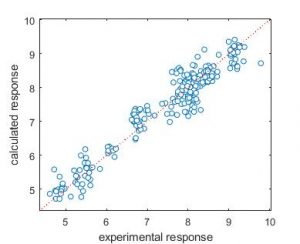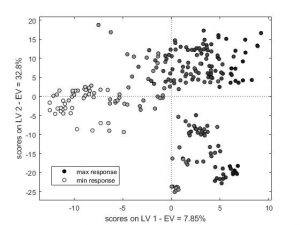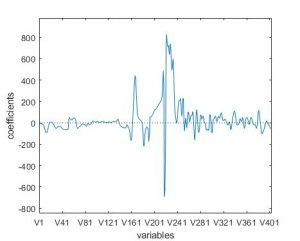## Regression toolbox for MATLAB now available!The Regression toolbox (for MATLAB) is a collection of MATLAB modules for calculating regression multivariate models: Ordinary Least Squares (OLS), Partial Least Squares (PLS), Principal Component Regression (PCR), Ridge regression, local regression based on K Nearest Neighbours (KNN) and Binned Nearest Neighbours (BNN) approaches, and variable selection approaches (All Subset Models, Forward selection, Genetic Algorithms and Reshaped Sequential Replacement). The toolbox is freely available and can be downloaded here

## QSAR for REACHMilano Chemometrics has been involved in several projects related to the use of QSAR for the REACH registration of chemicals. If you are interested in collaboration on these topics, read here.

## Molecular Descriptors Data BaseThe MOLE db – Molecular Descriptors Data Base is a free on-line database constituted of 1124 molecular descriptors calculated on 234773 molecules, released by Milano Chemometrics and QSAR Research Group: explore the MOLE db data base here!Visit the download page, where you can find softwares, MATLAB toolboxes and datasets you can freely download: N3-BNN toolbox, PCA toolbox, Kohonen and CPANN toolbox, Classification toolbox, Applicability Domain toolbox, RSR toolbox for variable selection.